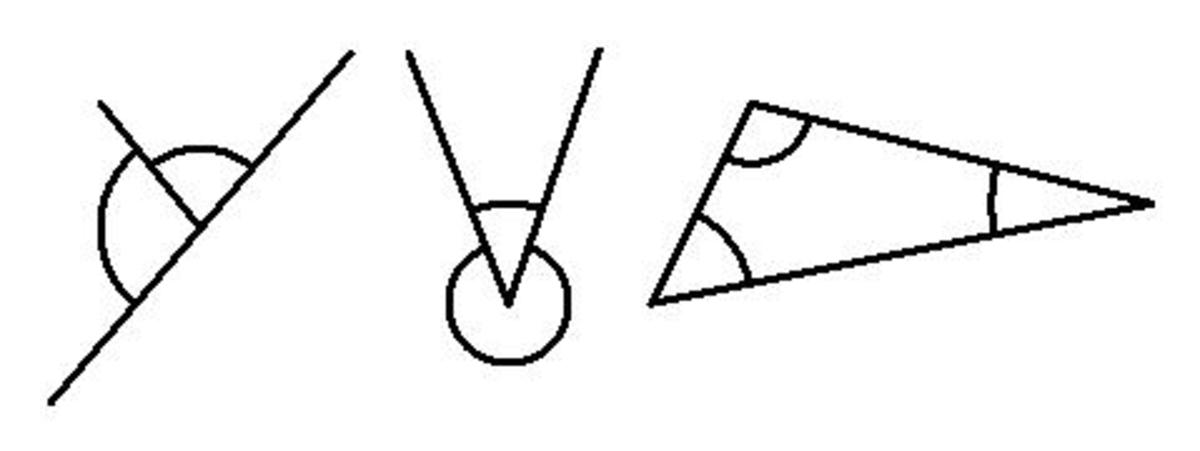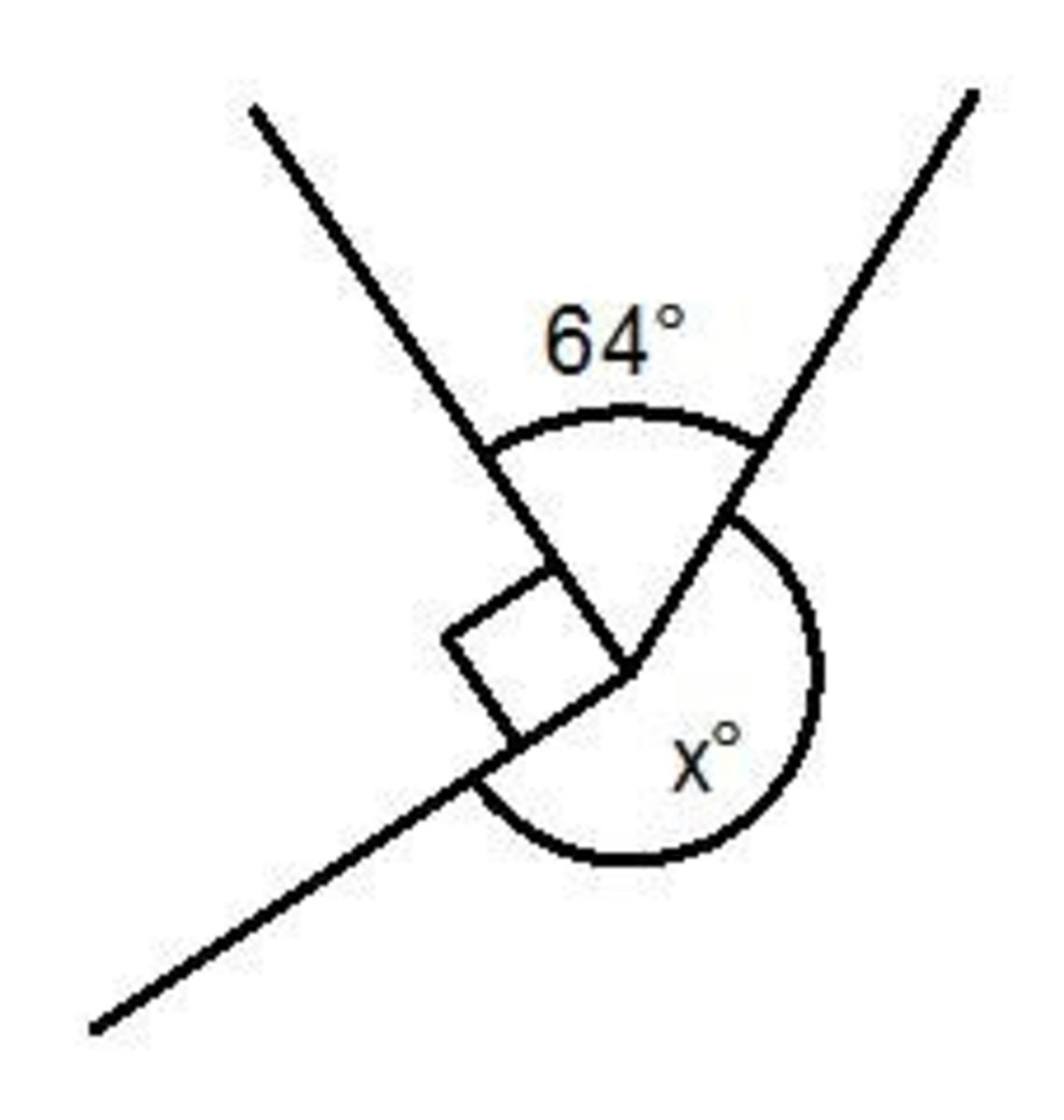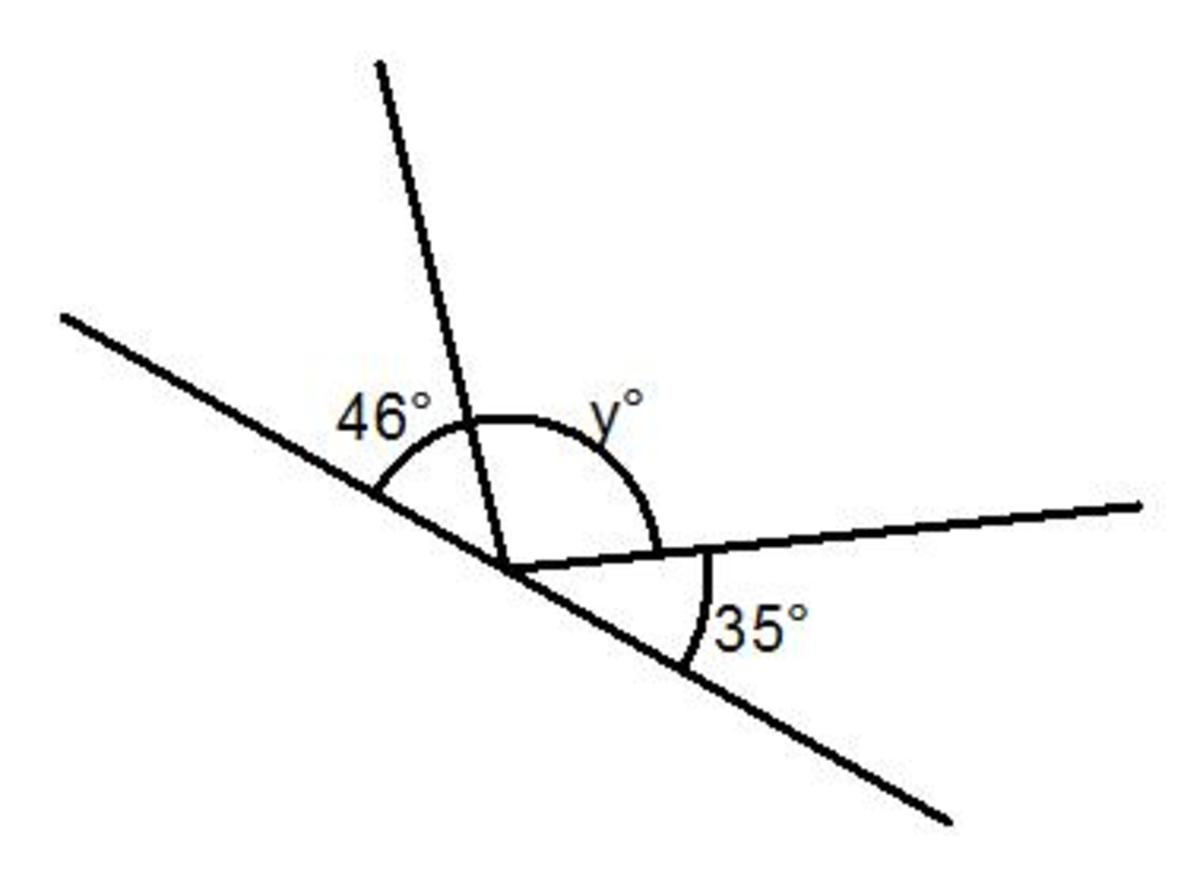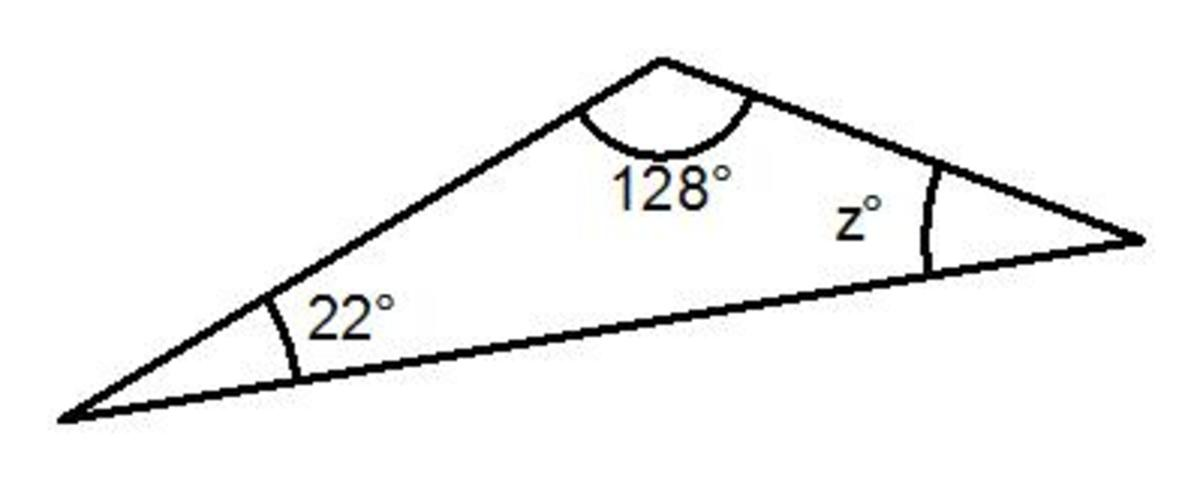Updated date:

# Basic math angle facts; angles in a triangle, on a straight line and around a point.

Author:There are 3 main angle facts to get to grips with in your math exams:

1) Angles on a straight line add up to 180⁰ (also known as angles in a half circle)

2) Angle around a point add up to 360⁰ (also known as angles in a full circle)

3) Angles in a triangle add up to 180⁰

So to solve a basic angle fact question you will first have to decide which angle fact to use. Then you need to add up the given angles and subtract this answer either 180⁰ or 360⁰ (depending on the angle fact you have used).

Let’s take a look at 3 examples that involve using these 3 basic angle facts that you get in many math tests.

Example 1

Work out the missing angle x.The angle fact you will need to use is angles around a point add up to 360⁰ (since the angles join up to make a full circle).

Next add up the angles that you are given. Make sure that you include the right angle in your total (a right angle is 90⁰):

64 + 90 = 154⁰

Next subtract this answer from 360⁰ to give you the size of angle x:

360 – 154 = 206⁰

x = 206⁰

You can see this angle is correct as the angle you were finding is a reflex angle (larger than 180⁰).

Example 2

Work out the missing angle y.The angle fact you will need to use is angles on a straight line add up to 180⁰ (since the angles form a half circle).

Next add up the angles that you are given:

46 + 35 = 81⁰

Next subtract this answer from 180⁰ to give you the size of angle y:

180 – 81 = 99⁰

y = 99⁰

Example 3

Work out the size of angle z.Since the shape is triangle, the angle fact is clearly angles in a triangle add up to 180⁰.

Like the last two angle questions first add up all of the angles in the triangle:

128 + 22 = 150⁰

Next subtract this answer from 180⁰ to give you the size of angle z:

180 – 150 = 30⁰

y = 30⁰

As you can see with these 3 examples the hardest part of the question is identifying the correct angle fact to use. Harder questions will involve applying more than one of the 3 angle facts listed above.

## Comments

Joan Whetzel on January 06, 2012:

Great descriptions and diagrams. I always love reading your math articles. They're informative and understandable for non-math people like me.